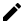# Make Text Match Any Background ColorHave you ever needed to use text inside a card that has generated colors? Give the user the capacity to choose their own color for a component that contains text like a navbar? These are situations where texts need to adapt to the current background color. Making each color match the text would be tedious and unscalable. By using the luminance and contrast ratio, we can determine if the text should be dark or bright while guaranteeing an accessible contrast.

## Contrast Ratio

The goal of text with a background is that it can be easily read by the users, including those with moderately low vision. To understand when a text is legible or not, first, we have to understand what contrast ratio is. Contrast ratio is a property that is defined by the luminance (amount of light) of the brightest color (white) to that of the darkest color (black) that a system is capable of producing. According to the W3C, the formula is `(L1 + 0.05) / (L2 + 0.05)`, where

• L1 is the relative luminance of the lighter of the colors, and
• L2 is the relative luminance of the darker of the colors.

The luminance scale can go from 0.0-1.0. Black being 0.0 and white 1.0. You may notice that if we calculate the ratio with the extremes of the scale's interval `(1.0 + 0.05) / (0.0 + 0.05)` we would get 21, and if both are 0.0 then we get 1. This means that the ratios will range from 1 to 21. These are commonly written as 1:1 to 21:1.

The recommended contrast ratio between a text and a background should be at least 4.5:1.

## Determining Text Color Dynamically

Now that we know what contrast ratio we should aspire to. It is time to find a formula so that we can determine which color to use.

According to Mark Ransom, to determine if we need a darker or brighter color, we just need to compare the contrast of background color with white and black. If the contrast for black is higher then we use black, otherwise, we use white. We can represent this in Javascript the following way:

``````if ((L + 0.05) / (0.0 + 0.05) > (1.0 + 0.05) / (L + 0.05)){
use #000000
} else {
use #ffffff
}``````

If we simplify it:

``````if (L > Math.sqrt(1.05 * 0.05) - 0.05){
use #000000
} else {
use #ffffff
}``````

Now, All that is left is calculating the luminance!

## Calculating the Luminance

Getting the luminance is pretty straightforward if you have an RGB color. According to the W3C, the formula for luminance is `L = 0.2126 * R + 0.7152 * G + 0.0722 * B` where R, G and B are defined as:

• if RsRGB <= 0.03928 then R = RsRGB/12.92 else R = ((RsRGB+0.055)/1.055) ^ 2.4

• if GsRGB <= 0.03928 then G = GsRGB/12.92 else G = ((GsRGB+0.055)/1.055) ^ 2.4

• if BsRGB <= 0.03928 then B = BsRGB/12.92 else B = ((BsRGB+0.055)/1.055) ^ 2.4

In Javascript this would translate to:

``````/*
If color has the following format:
const rgbColor = {
r: 100,
g: 100,
b: 100
}
*/

// Extracted from Polished
function getLuminance(rgbColor){
const [r, g, b] = Object.keys(rgbColor).map(key => {
// Our color numbers represent a 8bit channel.
// The formula requires a sRGB channel which is defined by
// ColorChannelIn8bit / 255
const channel = rgbColor[key] / 255
return channel <= 0.03928
? channel / 12.92
: ((channel + 0.055) / 1.055) ** 2.4
})
return parseFloat((0.2126 * r + 0.7152 * g + 0.0722 * b).toFixed(3))
} ``````

Frequently, we don't have our colors in RGB, but in hex or color name. That is why I recommend checking out polished library which exposes a `getLuminance` function that returns the luminance given a hex or color name.

## Bringing It All Together

Now that we know how to determine the color's value using its luminance and comparing contrast ratios, we are ready to apply it to an app. For the sake of this post, I will display how to do this with do a quick possible scenario. We have a card in which the user can change the background color with a color picker. Our job is to select a text color that is accessible and visible to all our users.

Here is a sandbox with the finished product. Feel free to tinker with it!

## Conclusion

Adjusting the text color to match a background is very common when developing dynamic UIs. A contrast ratio of 4.5:1 is essential to guarantee an accessible contrast between a text and a background. By comparing the contrast ratios of both white and black using the background color's luminance, it is possible to efficiently determine the text color used with a background.Contact Me